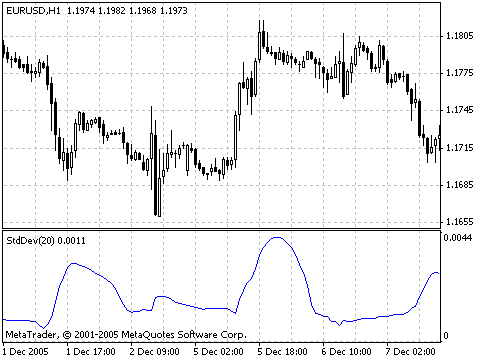Interesting script?
So post a link to it -
let others appraise it
You liked the script? Try it in the MetaTrader 5 terminal# Standard Deviation (StdDev) - indicator for MetaTrader 4

|
Views:
42296
Rating:
Published:
2005.12.20 15:38
Updated:
2016.11.22 07:32

The Standard Deviation Indicator (StdDev) measures the market volatility. This indicator describes the price standard deviation value relative to the Moving Average.

The higher the Standard Deviation is, the more instable (volatile) the market is, i.e., bars prices are rather dispersed relative to the moving average. Opposite, the lower the deviations is, the more immovable the market is, i.e., the bars prices are approaching to the moving average very much. It is known, however, that the market dynamics consists in interchange of quiet periods and activity spikes.

So, the approach to this indicator is simple:

• if the indicator value is too low (i.e., if the market is completely calm), it would be reasonable to expect the activity spike soon;

• on the contrary, if the indicator is extremely high, this means that the activity will slow down soon.

Calculation

StdDev (i) = SQRT (AMOUNT (j = i - N, i) / N)

AMOUNT (j = i - N, i) = SUM ((ApPRICE (j) - MA (ApPRICE (i), N, i)) ^ 2)

where:

• StdDev (i) — Standard Deviation of the current bar;
• SQRT — square root;
• AMOUNT(j = i - N, i) — sum of squares from j = i - N till i;
• N — smoothing period;
• ApPRICE (j) — the applied price of the j-th bar;
• MA (ApPRICE (i), N, i) — any moving average of the current bar for N periods;
• ApPRICE (i) — the applied price of the current bar.Full description of StdDev is available in the Technical analysis: Standard Deviation

Translated from Russian by MetaQuotes Ltd.
Original code: https://www.mql5.com/ru/code/8022Force Index (FRC)

Force Index (FRC) indicator measures the Bulls Power at each increase, and the Bulls Power at each decrease.Williams Percent Range

Williams’ Percent Range Indicator (%R) is a dynamic technical indicator, which determines whether the market is overbought/oversold.Money Flow Index (MFI)

Money Flow Index (MFI) is the indicator, which indicates the rate at which money is invested into a security and then withdrawn from it.Relative Vigor Index (RVI)

The main point of Relative Vigor Index Indicator (RVI) is that on the bull market the closing price is, as a rule, higher, than the opening price.